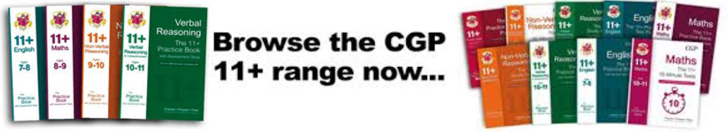Key Stage 2 Maths Objectives

Key Stage 2 - Year 5

· Multiply and divide any positive integer up to 10000 by 10 or 100 and understand the effect
· Order a given set of positive and negative integers
· Use decimal notation for tenths and hundredths
· Round a number with one or two decimal places to the nearest integer
· Relate fractions to division and to their decimal representations
· Calculate mentally a difference such as 8006 - 2993
· Carry out column addition and subtraction of positive integers less than 10000
· Know by heart all multiplication facts up to 10 x 10
· Carry out short multiplication and division of a three digit by a single digit integer
· Carry out long multiplication of a two digit by a two digit integer
· Understand area measured in square centimetres; understand and use the formula 'lxb' for area of a rectangle
· Recognise parallel and perpendicular lines, and properties of rectangles
· Use all four operations to solve simple word problems, involving numbers and quantities, including time

Key Stage 2 - Year 6

· Multiply and divide decimals mentally by 10 or 100, and integers by 1000, and   explain the effect
· Order a mixed set of numbers with up to three decimal places
· Reduce a fraction to its simplest form by cancelling common factors
· Use a fraction as an operator to find fractions of numbers or quantities
· Understand percentage as the number of parts in every 100
· Find simple percentages of small whole-number quantities
· Solve simple problems involving ratio and proportion
· Carry out column addition and subtraction of numbers involving decimals
· Derive quickly division facts corresponding to multiplication tables up to 10 x 10
· Carry out short multiplication and division of numbers involving decimals
· Carry out long multiplication of a three-digit by a two-digit integer
· Use a protractor to measure acute and obtuse angles to the nearest degree
· Calculate the perimeter and area of simple compound shapes that can be split into   rectangles